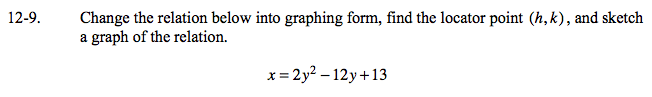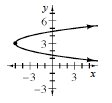### Home > A2C > Chapter 12 > Lesson 12.1.1 > Problem12-9

12-9.

Change the relation below into graphing form, find the locator point (h, k), and sketch a graph of the relation. Homework Help ✎ x = 2y2 − 12y + 13Complete the square of y.

x = 2(y2 − 6y) + 13

x = 2(y2 − 6y + 9) − 18 + 13
x = 2(y − 3)3 − 5

Locator point: (−5, 3)